# Maharashtra Board Class 5 Maths Solutions Chapter 12 Perimeter and Area Problem Set 48

Balbharti Maharashtra Board Class 5 Maths Solutions Chapter 12 Perimeter and Area Problem Set 48 Textbook Exercise Important Questions and Answers.

## Maharashtra State Board Class 5 Maths Solutions Chapter 12 Perimeter and Area Problem Set 48

Question 1.
Write the perimeter of each figure in the box given below it.

(1)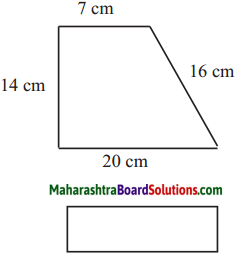Solution:
Perimeter [ ] DABCD
= 20 + 16 + 7 + 14
= 57 cm

∴ 57 cm(2)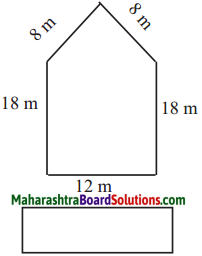Solution:
Perimeter of the figure
= 12 + 18 + 8 + 8 + 18
= 64m

∴ 64m

(3)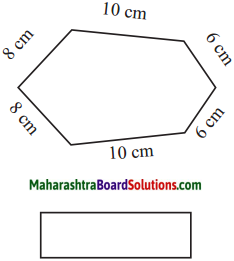Solution:
Perimeter of the figure
= 10 + 6 + 6 + 10 + 8 + 8
= 48 cm

∴ 48 cmQuestion 2.
If a square of side 1 cm is cut out of the corner of a larger square with side 3 cm (see the figure), what will be the perimeter of the remaining shape?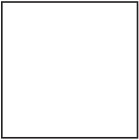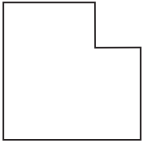Solution: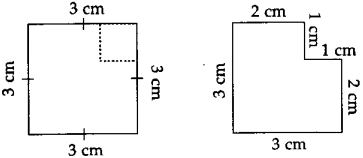Perimeter
= 2 + 3 + 3 + 2 + 1 + 1
= 12 cm

∴ 12 cm

Formula for the perimeter of a rectangle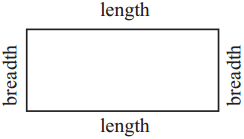Perimeter = length + breadth + length + breadth Opposite sides of a rectangle are of the same length.
So, the perimeter of a rectangle
= twice the length + twice the breadth
= 2 × length + 2 × breadthPerimeter of a rectangle = 2 × length + 2 × breadth

Example : The length of the rectangle below is 7 cm and its breadth, 3 cm. Let us find its perimeter.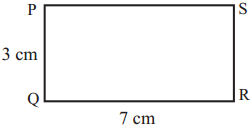Perimeter of rectangle PQRS = 2 × length + 2 × breadth
= 2 × 7 + 2 × 3
= 14 + 6
= 20
Therefore, the perimeter of the rectangle is 20 cm.

Formula for the perimeter of a square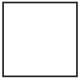The lengths of all the sides of a square are equal. Therefore, the perimeter of a square = four times the length of one of its sides.

Perimeter of a square = 4 × the length of one side

Example : The length of one side of a square is 6 cm. Find its perimeter. The perimeter of a square is four times the length of one side.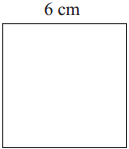Perimeter of a square = 4 × length of one side
= 4 × 6
= 24Therefore, the perimeter of the square is 24 cm.

Word problems

Example (1) The length of a rectangular park is 100 m, while its width is 80 m. What is its perimeter?

Perimeter of the rectangle = 2 × length + 2 × breadth
= 2 × 100 + 2 × 80
= 200 + 160
= 360

The perimeter of the rectangular park is 360 m.

Example (2) How much wire will be needed to put a triple fence around a square plot with side 30 m? What will be the total cost of the wire at ₹ 70 per metre ?

To put a single fence around the square plot, we need to find its perimeter.

Perimeter of a square = 4 × length of one side = 4 × 30 = 120

The perimeter of the square plot is 120 metres. Since the fence is to be a triple fence, we must triple the perimeter.

120 × 3 = 360 m of wire will be needed.

Now let us find out how much the wire will cost. One metre of wire costs ₹ 70.

Therefore, the cost of 360 m of wire will be 360 × 70 = 25, 200.The total cost of wire for putting a triple fence around the plot will be ₹ 25, 200.

Question 1.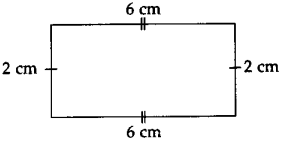Solution:
Perimeter of the figure
= 2 + 6 + 2 + 6
= 16 cm

∴ 16 cm

Question 2.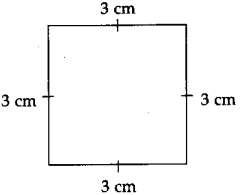Solution:
Perimeter of the figure
= 3 + 3 + 3 + 3
= 12 cm

∴ 12 cmQuestion 3.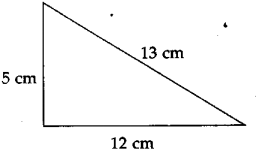Solution:
Perimeter of the figure
= 12 + 13 + 5
= 30 cm

∴ 30 cm

Question 4.
If four squares of side 1 cm ¡s cut out of all the corners of a larger square with side 4 cm (see the figure), what will be the perimeter of the remaining shape?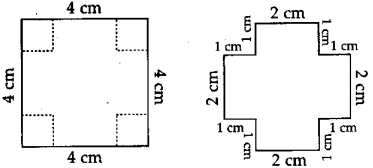Solution:
Perimeter
= 2 + 1 + 1 + 2 + 1 + 1 + 2 + 1 + 1 + 2 + 1 + 1
= 16 cm

∴ 16 cm